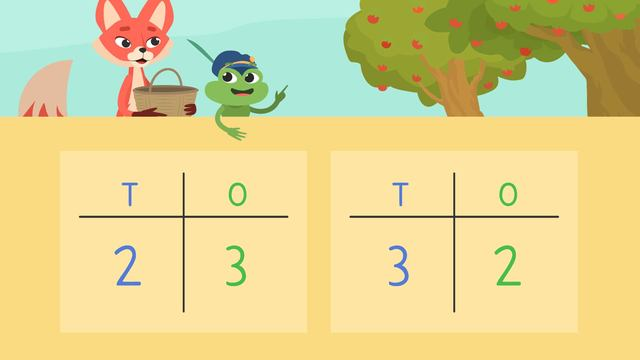# Comparing Numbers Up to 100Rate this video

Be the first to rate this video!

The authorTeam Digital

## Information about the videoComparing Numbers Up to 100

### Contents

#### Comparing Numbers Up to 100 What does comparing numbers mean? Comparing numbers in 1st grade means using base 10 blocks to be able to compare numbers two- two digits numbers and describe which one has more, less or the same. The comparing numbers first grade standard states the students will be able to compare 2 digit numbers based on the meanings of the tens and ones digits.

#### In this Video

In this comparing numbers video, Skylar and Henry decide to have an apple picking contest. They are going to count the apples on two different trees and guess which one has more apples. Whoever guesses incorrectly will have to climb up in the tree and pick all the apples. Who will win?

#### Comparing Numbers Examples

When comparing numbers 1st grade students look at the tens place first to see which digit is greater. If the tens place has the same digit, then we need to look at the digits in the ones place.

#### Follow Up Activities for Comparing Numbers to 100

There is additional comparing numbers for kids activities with comparing numbers worksheets, specifically, comparing numbers using place value worksheets.

### TranscriptComparing Numbers Up to 100

"Henry, let’s have an apple picking contest." "We'll count the apples on two trees then guess which one has more." "And if someone says the wrong number is greater, they have to climb up in the tree and pick ALL the apples." "DEAL!" "Comparing Numbers Up to 100" Tens and ones are PLACE VALUES. Each digit in a number has a place value. When digits are in the ONES place, they have the value of one each. When digits are in the tens place, they each have a value of ten. Skylar and Henry picked two trees and counted the apples. Henry counted twenty-three apples... and Skylar counted thirty-two. Henry says that twenty-three is greater, or bigger, than thirty-two and so HIS tree has more apples. Is Henry right ? (...) Let’s use a place value chart and base ten blocks to compare the numbers and see which one is GREATER. When COMPARING numbers, we start by looking at the TENS place and seeing which one has MORE. Let’s look at the numbers twenty-three and thirty-two. In the number twenty-three... the two is under the tens column because it's in the tens place… and tells us we need two tens. The three is under the ones because it's in the ONES place... and tells us we need three ones. Now, look at the number, thirty-two. The three is under the tens because it's in the TENS place. and the two is under the ones. How many TENS do we need to put under the tens place? (...) THREE. How many ONES do we put under the ones? (...) TWO Now we'll compare to see which number is greater. Next, we need to check the larger place value, or the TENS place, to see which number is BIGGER. HERE there is a two in the TENS place, which means we have twenty. In the number, thirty-two, there are THREE tens in the tens place, for thirty. There are more tens in thirty-two than twenty-three, so THIRTY-TWO is greater. Henry mixed up the place values and Skylar’s tree actually has more apples! "OH, (...) I GET IT!" "That was just practice." "Let’s try one more time." On the next try, Henry counted sixty-four apples on his tree… and Sklyar counted sixty-six apples. Skylar says she has counted more apples than Henry. Is Skylar right? (...) Let's put sixty- four and sixty-six in the place value charts. In the number sixty-four, how many tens do we have? (...) Six. How many do we put in the ones place? (...) Four. Look at the number sixty-six. How many tens do we have? (...) Six. How many do we put in the ones place? (...) Six. Now, we’ll compare to see which number is greater. Remember, we start with the tens place because it is the LARGER place value. What do you notice about the numbers in the tens place? (...) The numbers are the SAME. When the numbers are the same in the tens place, then we need to look at the ONES place to compare. Which number has more ones? (...) Sixty-six has more in the ones place, so it is GREATER than sixty-four. Skylar was right! While Skylar and Henry sort out the contest winner, (...)Remember… When COMPARING numbers, we start by looking at the TENS place to see which one has MORE. If the numbers are the same in the tens place, then we look at the ONES place to see which number is greater. "Wait! (...)Skylar(...) There are MORE apples on this tree than I counted!" " Wait Henry, (...) Let me help you!"